# Quick DimAngular Whole-Circle-Angle

## Recommended Postshey guys ,my 1st attempt in vanilla

It's just a quick whole-Circle dimension-Angular for line selection

Example: select 2 lines of 120° acute, you can get the rest 240° which >180°

(normal case command: _dimangular only get angle 60° which is

Its not the new topic but other threads show method

so just i share an alternative method for test.

```;hanhphuc@cadtutor acad2007

(defun c:test (/ l1 l2 m et1 et2 *error*)
(setvar "osmode" 0) ; bug if not zero
(setvar "cmdecho" 0)
(setq *cancel* *error*)
(defun *error* (msg)
(if
(or(= msg "Function cancelled")(= msg "quit / exit abort")
)
(princ "*Cancel*")
(princ (strcat "\n\; error: " msg ))
);if
(setq *error* *cancel*)
(princ)
)

(if  (and(setq l1 (car (entsel "\nPick line..")))
(=(setq et1 (cdr (assoc 0 (entget l1)))) "LINE"))
(progn
(if
(setq l2 (car (entsel "\nNext..")))
(setq et2 (cdr (assoc 0 (entget l2))))
(setq et2 et1 )
)
)
)
(cond
(
(= et1 "ARC" )
(command "_dimangular")  ;if not line, need to re-select ARC entity
)

(
(apply 'and
(mapcar '(lambda (x) (member x '("LINE"))) (list et1 et2))
) ;_  apply
(progn
(vl-remove-if '(lambda (x) (equal x (car m) 1e-5))
(setq m
(list (linterp l1 l2)
(midl l1)
(midl l2)
) ;_  list
) ;_  setq
) ;_  vl-remove-if
) ;_  progn
)

(t (command "_dimangular" "")); <vertex-1st-2nd angle point>
) ;_  cond

) ;_  defun

(defun[color="red"] flatz[/color] (_pt) ;
(if (and (vl-consp _pt) (= (length _pt) 3))
(reverse (cdr (reverse (mapcar 'float _pt))))
) ;_ end of if
) ;_ end of defun

(defun midp (a b / c d) ;get center
(mapcar '(lambda (c d) (* (+ c d) 0.5)) a b)
) ;_  defun

(defun midL (_entl /) ; mid point, by 2 line entity

(if (= (cdr (assoc 0 (entget _entl))) "LINE")

(apply 'midp (mapcar '(lambda (x) (flatz (cdr (assoc x (entget _entl))))) '(10 11)))

) ;_  if
) ;_  defun

(defun linterp (u v / lst) ; 2 Lines intersection 2D
(setq lst '(nil))
(apply 'inters
(foreach z (list u v)
(setq lst (append
(mapcar '(lambda (a b)
(flatz
(cdr (assoc a
(entget b)
) ;_  assoc
) ;_  cdr
) ;_  flatz
) ;_  lambda
'(10 11)
(list z z)
) ;_  mapcar
lst
) ;_  append
) ;_  setq
) ; foreach
) ;_  apply
) ;_  defun

```

hp#001

Edited by hanhphuc
flatz function updated

##### Share on other sites;dear guys, the 'if' logical in entsel normally should be this way:

(if

(and

(setq l1...)

(setq et1...)

(setq l2...)

(setq et2...)

)

;... T

;... Else

)

;the reason I didn't put in my code in order to skip selecting next entity if the 1st entity not a LINE.

If anybody has better practice is welcome to modify it, thank you##### Share on other sitesMultiple DimAngular on LWPolyline

;Works both closed & open LWPolyline

;can get 180° if vertex to vertex parallel;

;command: AngLWP

```
;Multiple DimAngular method in Visual Lisp
;hanhphuc  22/06/14
;v1.0
(defun c:AngLWP	(/ pl lst p1 p2 cc m en i v doc midp)
(setvar "cmdecho" 0)
(setq	*cancel* *error*
_oldos	 (getvar "osmode")
lst	 '() ; ** can not omit this
midp	 '((a b / c d) (mapcar '(lambda (c d) (/ (+ c d) 2.)) a b))
*error*	 '((msg)
(if
(wcmatch (strcase msg) "*CANCEL*,*QUIT*") ;"Function cancelled" "quit / exit abort"
(princ "*CANCEL*")
(princ (strcat "\nError: " msg))
)
(setq *error* *cancel*)
(princ)
)
) ;_ end of setq
(if (and (setq en (car (entsel "\nPick LWpolyline..")))
(equal (cdr (assoc 0 (entget en))) "LWPOLYLINE")
(setq v (cdr (assoc 90 (entget en)))
i -1
) ;_ end of setq
) ;_ end of and
(progn
(repeat v (setq pl (cons (vlax-curve-getPointAtParam en (setq i (1+ i))) pl)))
(if (= (cdr (assoc 70 (entget en))) 1)
(setq pl (append pl (list (car pl) (cadr pl))))
) ;_ end of if
(vla-StartUndoMark (setq doc (hp:doc)))
(setvar "osmode" 0) ; Edit
(foreach x (mapcar '(lambda (pt) (trans pt 0 1)) pl)
(setq lst (cons x lst))
(if (>= (length lst) 3)
(progn
(setq m (midp p1 p2))

(vl-list* (vlax-get-property
doc
(if (= "Model" (getvar "CTAB"))
"modelspace"
"paperspace"
) ;_ end of if
) ;_ end of vlax-get-property
(mapcar '(lambda (p) (vlax-3d-point (trans p 1 0))) ;lambda
(list cc (midp p1 cc) (midp cc p2) (polar m (angle m cc) (* (distance m cc) 1.25)))
) ;_ end of mapcar
) ;_ end of vl-list*
) ;_ end of apply

) ;_ end of progn
) ;_ end of if
) ;_ end of foreach
(vla-EndUndoMark doc)
(vlax-release-object doc)
) ;_ end of progn
) ;_ end of if
(setvar "osmode" _oldos); EDIT
(princ)
) ;_ end of defun

(defun-q-list-set 'hp:doc '(nil (vla-get-ActiveDocument (vlax-get-acad-object)))) ;inspired by LM:acdoc :-)

```

Edited by hanhphuc

##### Share on other sites`(defun-q-list-set 'hp:doc '(nil (vla-get-ActiveDocument (vlax-get-acad-object)))) ;inspired by LM:acdoc :-)`

Many thanks for the nod, though note that your function hp:doc is no different to writing (only defined as a list):

`(defun hp:doc nil (vla-get-activedocument (vlax-get-acad-object)))`

As the expression: (vla-get-activedocument (vlax-get-acad-object)) will be evaluated for every call of the hp:doc function - hence, there is no gain in efficiency as with LM:acdoc (where the active document object is only retrieved once).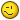##### Share on other sitesMany thanks for the nod, though note that your function hp:doc is no different to writing (only defined as a list):

`(defun hp:doc nil (vla-get-activedocument (vlax-get-acad-object)))`

As the expression: (vla-get-activedocument (vlax-get-acad-object)) will be evaluated for every call of the hp:doc function - hence, there is no gain in efficiency as with LM:acdoc (where the active document object is only retrieved once).```ya the only difference is with normal defun, returns: #<USUBR @2042d9d8 HP:DOC>
```

however, LM:acdoc gains its uniqueness!##### Share on other sitesjust noticed

(setvar "osmode" _oldos)

shouldn't put inside "foreach"...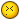so, code just updated.

##### Share on other sitesHello hanhphuc,

The code works like a charm!! Thanks !!

It would still be of great help if by some change in the code, we can annotate complementary angles (e.g by this code if some angle is shown as 90 degrees, the complementary angle would be 360-90= 270 Degrees)

I think it is possible with the code as we just need to reverse the angle measurement, but I have no clue as to how it can be done..

Can you help?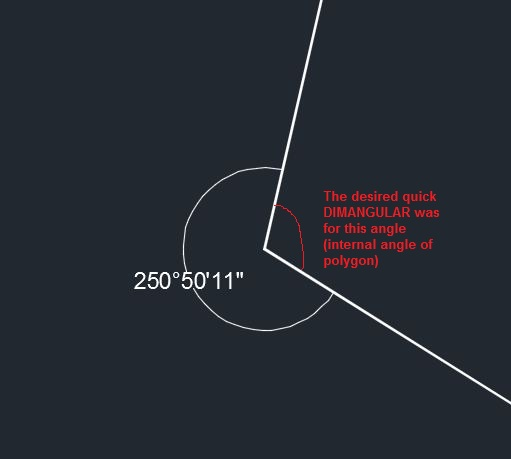Edited by torn_apart

##### Share on other sites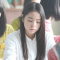Many thanks ,my friend hanhphuc ,

but ,I can't understand. can you make a demo ?

##### Share on other sitesHello hanhphuc,

The code works like a charm!! Thanks !!

It would still be of great help if by some change in the code, we can annotate complementary angles (e.g by this code if some angle is shown as 90 degrees, the complementary angle would be 360-90= 270 Degrees)

I think it is possible with the code as we just need to reverse the angle measurement, but I have no clue as to how it can be done..

Can you help?

hi torn_apart

welcome to the forum!

i not really understand, do you mean acute angle? but you can actually drag the to opposite side which >270

##### Share on other sitesHello hanhphuc,

Thanks for quick reply and warm welcome..

I get that, dragging it to the opposite side will give me the opposite angle.

But there are 100s of them...out of which the code is showing correct internal angles for 60...for the rest 40 this reversing would be needed...so I thought if we just reverse the angle measuring mechanism of the code and run it over the same set..then we would get correct angles for 40 and wrong for 60..

Then we can just merge the correct ones to get the whole thing.

##### Share on other sitesHello hanhphuc,

Thanks for quick reply and warm welcome..

I get that, dragging it to the opposite side will give me the opposite angle.

But there are 100s of them...out of which the code is showing correct internal angles for 60...for the rest 40 this reversing would be needed...so I thought if we just reverse the angle measuring mechanism of the code and run it over the same set..then we would get correct angles for 40 and wrong for 60..

Then we can just merge the correct ones to get the whole thing.

The code put the larger angle >180, so what you mean put acute angle as well?

##### Share on other sitesYes

Only smaller angles.

##### Share on other sitesYes

Only smaller angles.

updated v1.1

```

;Multiple DimAngular method in Visual Lisp
;AngLwp.lsp
;v1.0: 22/06/14
;v1.1: 10/12/2014
;	*dimsuppressed* dimension suppressed, only dimension text visible
;	*dimtxtinside* dimension text positin inside / outside
;	*error* localize & optimized
;	text placement works in UCS

; users can adjust global settings to suit their need here ,[color="red"] t / nil[/color]
[color="blue"]
(setq
*dimsuppressed* [color="red"][b]nil[/b] [/color] ; t= suppressed / [b][u]nil[/u]= normal [/b]
*dimtxtinside* [color="red"][b]t [/b][/color] ; [b][u]t[/u]= acute angle[/b] / nil= obtuse large > 180
)[/color]

(defun c:AngLWP	( / *error* pl lst p1 p2 cc m en l i v doc midp var sz box vobj )
;hanhphuc 10/12/2014 anglwp.lsp (v1.1)
(setq	l   '("cmdecho" "osmode" )
var  (mapcar 'getvar l)
sz	(getvar "dimtxt")
lst	 '() ; ** can not omit this
midp	 '((a b / c d) (mapcar '(lambda (c d) (/ (+ c d) 2.)) a b)));_ end of setq

(mapcar 'setvar l '(0 0 ))

(defun *error* (msg)
(if (= 8 (logand 8 (getvar "undoctl")))
(vla-EndUndoMark (hp:doc))
) ;_ end of if
(if doc
(vlax-release-object doc)
) ;_ end of if
(if (wcmatch (strcase msg) "*CANCEL*,*QUIT*,*BREAK*")
(princ (strcat "\nError: " msg))
) ;_ end of if
(mapcar 'setvar l var)
(princ)
) ;_ end of defun

(if (and (setq en (car (entsel "\nPick LWpolyline..")))
(eq (cdr (assoc 0 (entget en))) "LWPOLYLINE")
(setq v (cdr (assoc 90 (entget en)))
i -1
) ;_ end of setq
) ;_ end of and
(progn
(repeat v (setq pl (cons (vlax-curve-getPointAtParam en (setq i (1+ i))) pl)))
(if (= (cdr (assoc 70 (entget en))) 1)
(setq pl (append pl (list (car pl) (cadr pl))))
) ;_ end of if
(vla-StartUndoMark (setq doc (hp:doc)))
(foreach x (mapcar '(lambda (pt) (trans pt 0 1)) pl)
(setq lst (cons x lst))
(if (>= (length lst) 3)
(progn
(setq m    (midp p1 p2)
vobj (apply
(vl-list* (vlax-get-property
doc
(if (= "Model" (getvar "CTAB"))
"modelspace"
"paperspace"
) ;_ end of if
) ;_ end of vlax-get-property
(mapcar '(lambda (p) (vlax-3d-point (trans p 1 0)))
(list cc (midp p1 cc) (midp cc p2) (polar m (angle m cc) (* (distance m cc) (if *dimtxtinside* 0.5 1.25 ))))
) ;_ end of mapcar
) ;_ end of vl-list*
) ;_ end of apply
) ;_ end of setq

(if  *dimsuppressed* (progn
((lambda (obj)
(mapcar
'(lambda (a b) (vlax-put obj a b))
"TextHeight"		 "VerticalTextPosition"   "TextGap"	"TextInside"
"DimLine1Suppress"   "DimLine2Suppress" ;<--- "line" visibility, thanks marko :-)
"ExtLine1Suppress" "ExtLine2Suppress"
"TextInsideAlign" "TextOutsideAlign"
)
(list 19 19 0. 0. sz (if (LM:ListClockwise-p pl) 1 2) sz 1 1 1 1 1 0 0)
)
(vlax-put-property obj 'TextFill :vlax-true)  ;<-- text masked:  1 / 0
)
vobj
) ;_ end of lambda

(command "_explode"  (vlax-vla-object->ename vobj))
(setq rot(cdr(assoc 50 (entget(entlast)))))
(command "_U")
(vla-put-TextRotation (vlax-ename->vla-object (entlast))
(atan (apply '/ (cdr (reverse (getvar "ucsxdir" ))))))))
)
)
) ;_ end of progn
) ;_ end of if

) ;_ end of foreach
(vla-EndUndoMark doc)
(vlax-release-object doc)
) ;_ end of progn
) ;_ end of if
(mapcar 'setvar l var ); EDIT
(princ)
) ;_ end of defun

(defun-q-list-set 'hp:doc '(nil (vla-get-ActiveDocument (vlax-get-acad-object)))) ;inspired by LM:acdoc :-)
(princ "\nMultiple DimAngular on polygon. command: anglwp")(princ)

;; coutesy & reference
;; List Clockwise-p - Lee Mac
;; Returns T if the point list is clockwise oriented
http://www.lee-mac.com/mathematicalfunctions.html
(defun LM:ListClockwise-p ( lst )
(minusp
(apply '+
(mapcar
(function
(lambda ( a b )
)
)
lst (cons (last lst) lst)
)
)
)
)

;; Make Angle Readable by: ymg
(setq a (rem (+ a pi pi) (+ pi pi)))
(rem (if (< (* pi 0.5) a (* pi 1.5))(+ a pi) a) (+ pi pi))
)

```

Edited by hanhphuc
updated v1.1

##### Share on other sitesThanks hanhphuc,

That worked...

I am a bit new to lisp and autocad, if you can please explain me a bit about the code (and the logic behind it) which you have written, it would be of much help and would also help me in writing a few of my own and thereby helping people.

Thanks again

##### Share on other sitesThanks hanhphuc,

That worked...

I am a bit new to lisp and autocad, if you can please explain me a bit about the code (and the logic behind it) which you have written, it would be of much help and would also help me in writing a few of my own and thereby helping people.

Thanks again

im not good explain, dimension was created using activeX method vla-AddDim3PointAngular which refered to developer documentations.

same as manually command requires arguments (in red)

see inside the code :

(list cc (midp p1 cc) (midp cc p2) (polar m (angle m cc) (* (distance m cc) 1.25))) ;

AngleVertex = cc

FirstEndPoint = (midp p1 cc)

SecondEndPoint = (midp cc p2)

TextPoint = (polar m (angle m cc) (* (distance m cc) 1.25)) ;

;note: midp is the sub-function to obtain midpoint of 2 vertex

since you are new i just brief the method:

1.collect the coordinates of lwpolyline's vertex, check closed or not.

2.get the midpoint each leg for FirstEndPoint, SecondEndPoint

3.collect every 3 points AngleVertex , FirstEndPoint , SecondEndPoint respectively

4.using polar to obtain TextPoint

5.repeat the loop using foreach function

when you do manually almost same, the difference is just we need to automate determining every 3-vertex

HTH##### Share on other sitesI know , this is dim ang.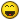But, is time to rest ,my friend , Don't be too hard !## Join the conversation

You can post now and register later. If you have an account, sign in now to post with your account.
Note: Your post will require moderator approval before it will be visible.×   Pasted as rich text.   Paste as plain text instead

Only 75 emoji are allowed.